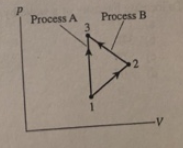# Problem: Two process are shown that take an ideal gas from state 1 to state 3. Compare the work done by process A to Process A the work done by process B. a) WA &gt; WBb) WA &lt; WB c) WA =WB = 0d) WA = WB but neither is zero

###### FREE Expert Solution

Work is only done when the volume of the gas changes.

Therefore, a PV diagram gives a visual interpretation of work done.

82% (144 ratings)###### Problem Details

Two process are shown that take an ideal gas from state 1 to state 3. Compare the work done by process A to Process A the work done by process B.a) WA > WB

b) WA < WB

c) WA =WB = 0

d) WA = WB but neither is zero